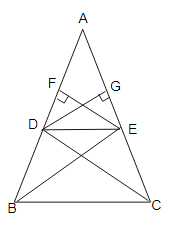# Prove that if a line is drawn parallel to one side of a triangle to intersect the other two sides, then the two sides are divided in the same ratio.

To do:

We have to prove that if a line is drawn parallel to one side of a triangle to intersect the other two sides, then the two sides are divided in the same ratio.

Solution:Let a $\triangle \mathrm{ABC}$ in which a line $\mathrm{DE}$ parallel to $\mathrm{BC}$ intersects $\mathrm{AB}$ at $\mathrm{D}$ and $\mathrm{AC}$ at $\mathrm{E}$.

Construction: Join $B E, C D$ and draw $E F \perp A B$ and $D G \perp A C$

$\frac{\operatorname{ar}(\triangle A D E)}{\operatorname{ar}(\triangle B D E)}=\frac{\frac{1}{2} \times A D \times E F}{\frac{1}{2} \times D B \times E F}$

$=\frac{A D}{D B}$.........(i)

Similarly,

$\frac{\operatorname{ar}(\triangle A D E)}{\operatorname{ar}(\triangle D E C)}=\frac{\frac{1}{2} \times A E \times G D}{\frac{1}{2} \times E C \times G D}$

$=\frac{A E}{E C}$.............(ii)

$\triangle B D E$ and $\triangle D E C$ lie between the same parallels $D E$ and $B C$ and on the same base $DE$.

Therefore,

$\operatorname{ar}(\triangle B D E)=\operatorname{ar}(\triangle D E C)$..........(iii)

From (i), (ii) and (iii), we get,

$\frac{A D}{D B}=\frac{A E}{E C}$

Hence proved.

Updated on: 10-Oct-2022

32 Views